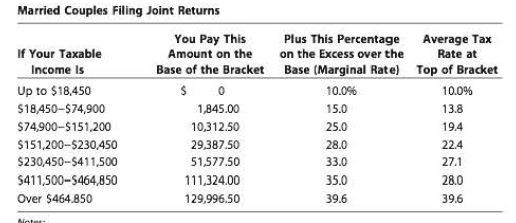Chapter 3, Problem 8PFundamentals of Financial Manageme...

15th Edition
Eugene F. Brigham + 1 other
ISBN: 9781337395250

Solutions

Chapter
SectionFundamentals of Financial Manageme...

15th Edition
Eugene F. Brigham + 1 other
ISBN: 9781337395250
Textbook Problem

PERSONAL TAXES Susan and Stan Britton are a married couple who file a joint income tax return, where the tax rates an? based on the tax tables presented in the chapter. Assume that their taxable income this year was $375,000. a. What is their federal tax liability? b. What is their marginal tax rate? c. What is their average tax rate?a. Summary Introduction To compute: The federal tax liability. Federal Tax Liability: The obligation of an individual or a company towards the government based on the earnings during a specified period is called federal tax liability. It is calculated as per the predetermined rates of tax. Explanation Given, The filling status is married and filling jointly. Annual taxable income is$375,000.

Tax slab is bracket five (refer the table in the chapter).

Base bracket is $230,450-$411,500.

Base tax is $51,577.50. Formula to compute the federal tax liability, Federal Tax Liability=Base Tax+(Taxable IncomeBase) Substitute$51,577.5 for base tax, $375,000 for taxable income and$230,450 for base.

Federal Tax Liability=\$51,577

b.

Summary Introduction

To identify: The marginal tax rate.

Marginal Tax Rate:

The marginal tax rate is the rate that is calculated on the upper limit of the taxable income. It computes the tax liability over and above the base tax.

c.

Summary Introduction

To compute: The average tax rate.

Average Tax Rate:

The average tax rate is the rate that is eventually paid on the total taxable income. It is computed by dividing total tax liability and the taxable income.

Still sussing out bartleby?

Check out a sample textbook solution.

See a sample solution

The Solution to Your Study Problems

Bartleby provides explanations to thousands of textbook problems written by our experts, many with advanced degrees!

Get Started

Describe the major components of a TQM program.

Foundations of Business (MindTap Course List)

Explain how absolute advantage and comparative advantage differ.

Principles of Microeconomics (MindTap Course List)

What are some pros and cons of holding high levels of current assets in relation to sales? Use the DuPont equat...

Fundamentals of Financial Management, Concise Edition (with Thomson ONE - Business School Edition, 1 term (6 months) Printed Access Card) (MindTap Course List)